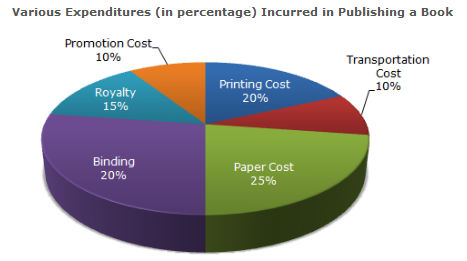Direction The following pie-chart shows the percentage distribution of the expenditure incurred in publishing a book. Study the pie-chart and the answer the questions based on it.1.

If for a certain quantity of books, the publisher has to pay Rs. 30,600 as printing cost, then what will be amount of royalty to be paid for these books?

 A. Rs 19,450 B. Rs 21,200 C. Rs 22,950 D. Rs 26,150
Explanation: Let the amount of Royalty to be paid for these books be Rs. r. Then, 20 : 15 = 30600 : r => r = Rs. (30600x15)/20 = Rs. 22,950.

2.

What is the central angle of the sector corresponding to the expenditure incurred on Royalty?

 A. 15º B. 24º C. 54º D. 48º
Explanation: Central angle corresponding to Royalty = (15% of 360)º = ( 15 x 360 ) º 100 = 54º.

3.

The price of the book is marked 20% above the C.P. If the marked price of the book is Rs. 180, then what is the cost of the paper used in a single copy of the book?

 A. Rs. 36 B. Rs. 37.50 C. Rs. 42 D. Rs. 44.25
Explanation: Clearly, marked price of the book = 120% of C.P. Also, cost of paper = 25% of C.P Let the cost of paper for a single book be Rs. n. Then, 120 : 25 = 180 : n => n = Rs. ( 25 x 180 ) = Rs. 37.50 .

4.

If 5500 copies are published and the transportation cost on them amounts to Rs. 82500, then what should be the selling price of the book so that the publisher can earn a profit of 25%?

 A. Rs. 187.50 B. Rs. 191.50 C. Rs. 175 D. Rs. 180